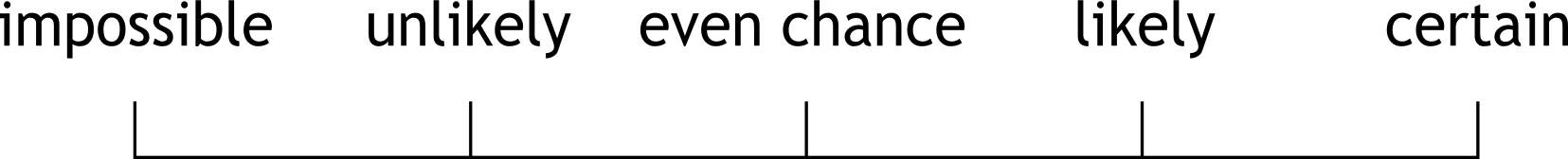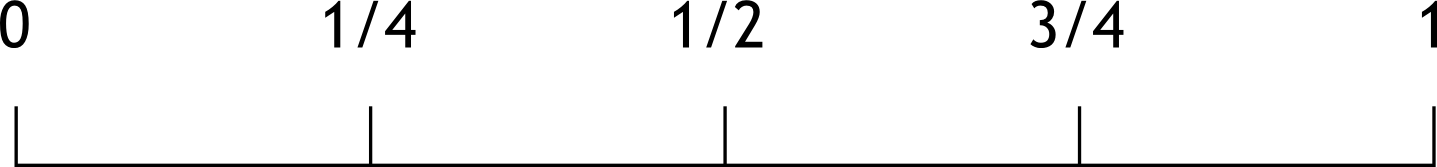The Singing Hedgehog Guide to:
Probability 1

Probability is all about chance or likelihood.
We can show chance on a probability scale:This is obviously vague so we normally use a numerical scale:Probability is mostly written as a fraction    1/2

sometimes as a percentage                         50%
rarely as a decimal                                     0.5
NEVER as a ratio                                        1:2
next page